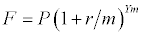A deterministic model is a model that gives you the same exact results for a particular set of inputs, no matter how many times you re-calculate it.

An example of a deterministic model is a calculation to determine the return on a 5-year investment with an annual interest rate of 7%, compounded monthly. The model is just the equation below:The inputs are the initial investment (P = \$1000), annual interest rate (r = 7% = 0.07), the compounding period (m = 12 months), and the number of years (Y = 5).

Compound Interest Model
Present value, P
Annual rate, r
Periods/Year, m
Years, Y
Future value, F

One of the purposes of a model such as this is to make predictions and try "What If?" scenarios. You can change the inputs and recalculate the model and you'll get a new answer. You might even want to plot a graph of the future value (F) vs. years (Y). In some cases, you may have a fixed interest rate, but what do you do if the interest rate is allowed to change? For this simple equation, you might only care to know a worst/best case scenario, where you calculate the future value based upon the lowest and highest interest rates that you might expect.